# Intrinsic flow equation

## Definition

### Definition with symbols

An intrinsic flow equation is a differential equation: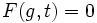$F(g,t)=0$

where$g$ is the metric tensor,$t$ isa time-parameter, and$F$ could involve taking any of the tensors arising naturally out of the metric tensor, and of course the various derivatives.

The interpretation of the intrinsic flow equation is as follows. Take any differential manifold$M$ and consider a Riemannian metric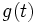$g(t)$ on$M$ that varies with$t$. This is a flow of the Riemannian metric on the differential manifold.

The flow satisfies the intrinsic flow equation if, for any point$p \in M$,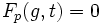$F_p(g,t) = 0$ where differentials with respect to$t$ are interpreted in the natural sense.

## Examples

### Ricci flow equation

Further information: Ricci flow

The Ricci flow equation is given by: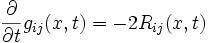$\frac{\partial}{\partial t} g_{ij}(x,t) = -2R_{ij} (x,t)$

The stationary points for the corresponding flow on any manifold are precisely the constant-curvature metrics.

### Volume-normalized Ricci flow equation

Further information: volume-normalized Ricci flow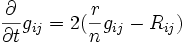$\frac{\partial}{\partial t} g_{ij} = 2 (\frac{r}{n} g_{ij} - R_{ij})$